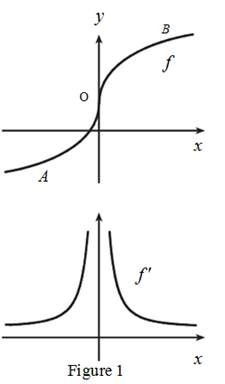# The graph of f ′ below the graph of f .### Single Variable Calculus: Concepts...

4th Edition
James Stewart
Publisher: Cengage Learning
ISBN: 9781337687805### Single Variable Calculus: Concepts...

4th Edition
James Stewart
Publisher: Cengage Learning
ISBN: 9781337687805

#### Solutions

Chapter 2.7, Problem 11E
To determine

## To Sketch: The graph of f′ below the graph of f.

Expert Solution

### Explanation of Solution

From the given graph, it is observed that the graph of f contains the vertical tangent at one point. Let this point be O.

At the point O, the derivative of f is infinity and the derivative curve has a vertical asymptote at that point.

From the given graph, it is observed that f has a positive slope.

This implies that, the derivative will be positive everywhere.

From the point A to left, the slope of the graph is decreasing gradually which implies that, the derivative graph must be decreasing towards zero.

From the point B towards right, the slope of the graph is decreasing gradually which implies that, the derivative graph must be decreasing towards zero.

Graph:

Use the above information and trace the graph of f(x) as shown below in Figure 1.Thus, f is the required graph.

### Have a homework question?

Subscribe to bartleby learn! Ask subject matter experts 30 homework questions each month. Plus, you’ll have access to millions of step-by-step textbook answers!